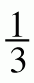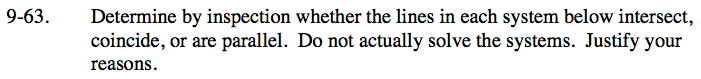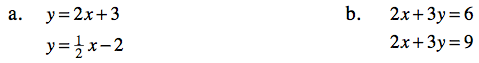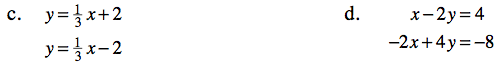Home > CC3 > Chapter 9 > Lesson 9.2.1 > Problem9-63

9-63.
1. Determine by inspection whether the lines in each system below intersect, coincide, or are parallel. Do not actually solve the systems. Justify your reasons. Homework Help ✎

1. y = 2x + 3
y =x − 2

2. 2x + 3y = 6
2x + 3y = 9

3. y =x + 2
y =x − 2

4. x − 2y = 4
−2x + 4y = −8Parallel lines have equal slope.
Lines that coincide have equal slope and equal y-intercepts.
Lines that neither coincide or are parallel will intersect once.The coefficient of x tells you the slope.
Are they the same?

These equations have the same coefficient for x and y, but have different constants. What does this tell you about that lines?Notice that the coefficients of x are the same in the two equations.

The lines are parallel.

Write both equations in y = mx + b form.

x − 2y = 4

${\it y} = \frac{1}{2}{\it x} - 2$

−2x + 4y = −8

${\it y} = \frac{1}{2}{\it x} - 2$

Because the two equations are equal, the lines coincide.